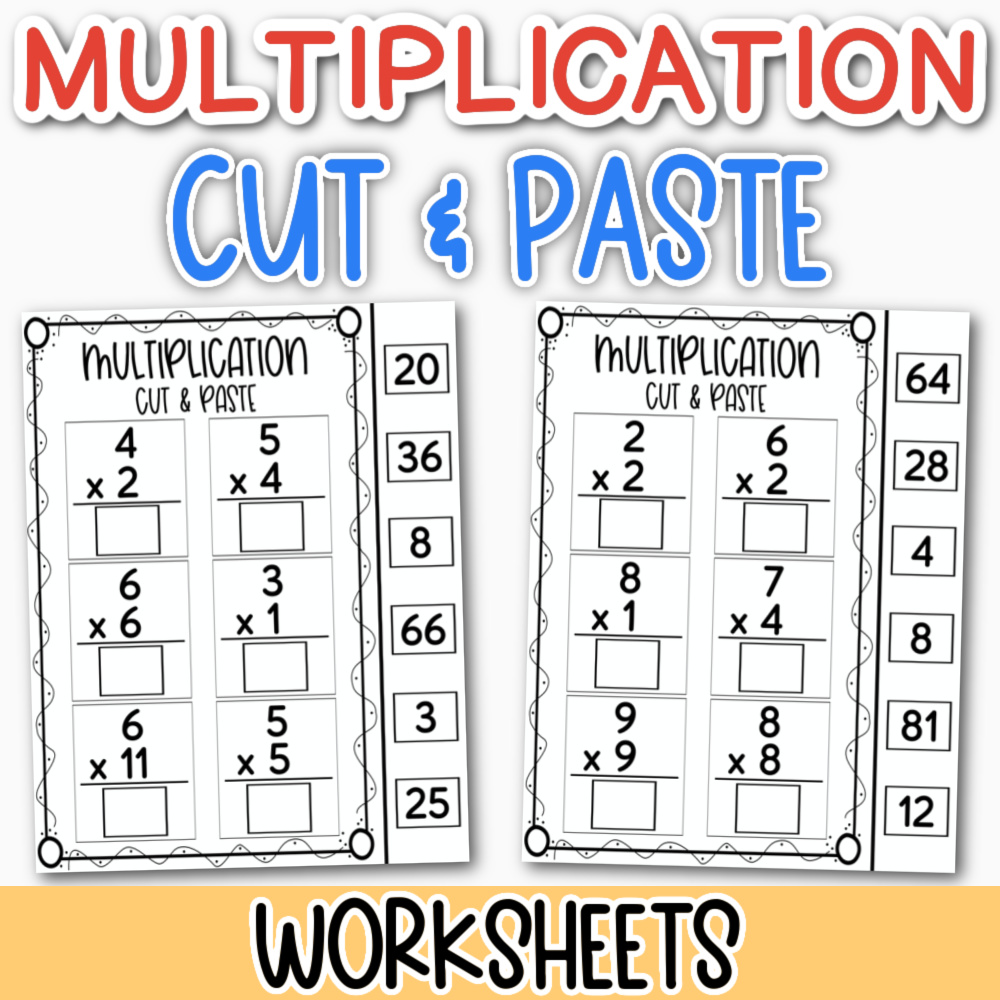# Multiplication Cut and Paste Worksheets | Free | Single and Double Digit

## Multiplication Cut and Paste

There are so many steps to teaching multiplication. It starts with understanding what multiplication is (area or arrays), moves into learning the math facts, and proceeds into conquering two digit and three digit multiplication.

There is a step that I often skipped as a classroom teacher….multiplication properties.

Mastering words like associate and distributive sounded hard, and took away from the time needed to learn those multiplication facts! Though the more I have learned about math, and teaching the concepts and not just formulas, I’m learning this is important too.

When we teach children the properties of multiplication, we are actually equipping them to solve numerous expressions. And, it even begins to build a foundation in algebra.

Important stuff, right?

So today, I have a few multiplication cut and paste activities to help your children learn these important properties.

## Cut and Paste Worksheets for Multiplication

These multiplication properties cut and paste activities are really no prep.

1. First, print off the pages you need on regular copy paper.
2. Then provide glue and scissors and you are ready to go.

### How to Use Multiplication Cut and Paste Activity

Before you pass out these multiplication properties cut and paste activities, it is important to understand what each word means.

### Communitive Property in Multiplication

This is probably the easiest of the three properties included in the cut and paste packet.

Just like addition, the answer does not change if you add 5 + 6 or 6 + 5. You can multiply 3 x 4 or 4 x 3 and you will get the answer twelve either way. A simple definition is that order does not matter.

In the Cut and Paste packet, there is only one page for this…..as it is pretty simple. The children simply find the multiplication expression that will make the equation true.

For example, the first question is 6 x 4 = ___________. The children must find 4 x 6 as this will make the equation true.

### Associative Property in Multiplication

As we move to our next multiplication property, things get a little more complicated. We are no longer multiplying two digits, we are multiplying three or more. But once again the order does not matter….or more specifically where we put the parenthesis is does not matter.

Let’s look at the problem 2 x 3 x 4.

I could solve the problem like this……

(2 x 3) x 4

6 x 4

24

or like this……

2 x (3 x 4)

2 x 12

24

As you can see, no matter where I put the parenthesis the answer is 24.

There are two cut and paste activity pages for this property. The first one has problems like 4 x 6 = _______. The children then have to find the expression that makes the equation true. In this case, the answer is 4 x (2 x 3). There are also some that just show the parenthesis switched. Like (8 x 5) x 15 = 8 x (5 x 15)

The other cut and paste activity has the children just solving expression where they could use the associated property to solve.

### Distributive Property in Multiplication

Now, this is a fun one. Distributive property basically says that you can either multiply the sum of two digits or multiply those two digits separately.

For example, in the problem 5 (3 + 2) I could do two things…..

I could multiply the five times the 3 and then times the 2.

5 x 3 + 5 x 2

15 + 10

25

or I could add up the addends first.

5 (3 + 2)

5 x 5

25

There are also two cut and paste activities for this property. The first one has the children once again making equation true.

They may be asked to find the equivalent expression to 9 (5 + 2) which would be (9 x 5) + (9 x 2). Sometimes, they may be given (2 x 3) + (2 x 5) which of course the equivalent expression would be 2 (3 + 5).

Enjoying playing with these different multiple properties.

## Free Cut and Paste Multiplication Worksheets

Here you go, hit the arrow to download and print what you need from these multiplication cut and paste activities.

MultiplicationCutPaste

You’ve Got This!